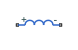# Inductor

Linear inductor in electrical systems

• Library:
• Simscape / Foundation Library / Electrical / Electrical Elements

•## Description

The Inductor block models a linear inductor, described with the following equation:

`$V=L\frac{dI}{dt}$`

where:

• V is voltage.

• L is inductance.

• I is current.

• t is time.

The Series resistance and Parallel conductance parameters represent small parasitic effects. The series resistance can be used to represent the DC winding resistance or the resistance due to the skin effect. Simulation of some circuits may require the presence of a small parallel conductance. For more information, see Modeling Best Practices.

Connections + and – are conserving electrical ports corresponding to the positive and negative terminals of the inductor, respectively. The current is positive if it flows from positive to negative, and the voltage across the inductor is equal to the difference between the voltage at the positive and the negative terminal, V(+) – V(–).

### Variables

To set the priority and initial target values for the block variables prior to simulation, use the Initial Targets section in the block dialog box or Property Inspector. For more information, see Set Priority and Initial Target for Block Variables.

Nominal values provide a way to specify the expected magnitude of a variable in a model. Using system scaling based on nominal values increases the simulation robustness. Nominal values can come from different sources, one of which is the Nominal Values section in the block dialog box or Property Inspector. For more information, see Modify Nominal Values for a Block Variable.

## Ports

### Conserving

expand all

Electrical conserving port associated with the inductor positive terminal.

Electrical conserving port associated with the inductor negative terminal.

## Parameters

expand all

Inductance value.

Represents small parasitic effects. The series resistance can be used to represent the DC winding resistance or the resistance due to the skin effect.

Represents small parasitic effects. Simulation of some circuits may require the presence of a small parallel conductance.

## Version History

Introduced in R2007a